# v3. At what distance from a point charge of 4.0 nC does the electric field have...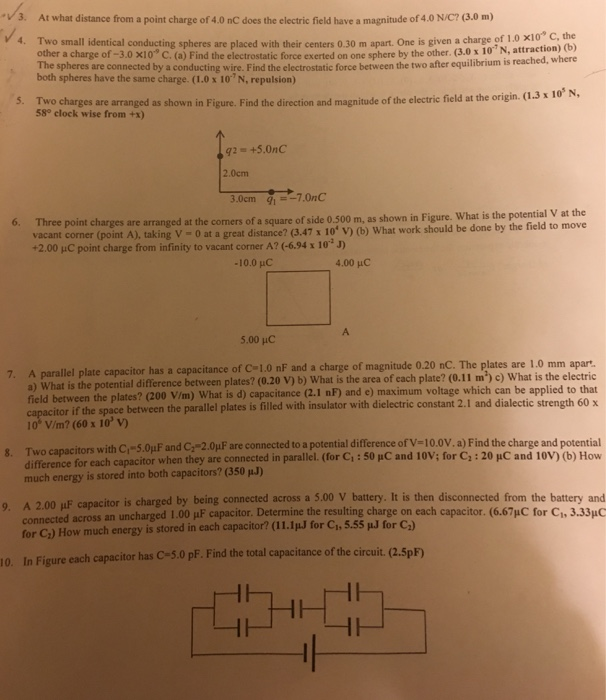v3. At what distance from a point charge of 4.0 nC does the electric field have a magnitude of 4.0 N/C? (3.0 m) 4. Two small identical conducting spheres are placed with their centers 0.30 m apart. One is given a charge of 1 0 x10* c other a charge of -3.0 x10 C. (a) Find the electrostatic force exerted on one sphere by the other. (3.0 x 10"N, attraction) the (b) e spheres are connected by a conducting wire. Find the electrostatic force between the two after equilibrium is rea both spheres have the same charge. (1.0x 10 N, repulsion) 5. Two charges are arranged as shown in Figure. Find the direction and magnitude of the electri field at the origin. (.3x10 58° clock wise from +x) 2.0cm 6. Three point charges are arranged at the comers of a square of side 0.500 m, as shown in Figure. What is the potential V at the vacant corner (point A), taking V -0 at a great distance? (3.47 x 10' v uC point charge from infinity to vacant corner A? 6.94 x 10 J) -10.0 HC 4.00 pHC 5.00 pC llel plate capacitor has a capacitance of C 1.0 nF and a charge of magnitude 0.20 nC. The plates are 1.0 mm apart a) What is the potential difference between plates? (0.20 V) b) What is the area of cach plate? (0.11 m') c) What is the electric field between the plates? (200 V/m) What is d) capacitance (2.1 nF) and e) maximum voltage which can be applied to that itor if the space between the parallel plates is filled with insulator with dielectric constant 2.1 and dialectic strength 60x 10° V/m? (60 x 10' v) T ca actors with C -5 0μF and C2-2OpFare connected to a potential difference ofV=10.0V.a) Find the charge and potential difference for each capacitor when they are connected in parallel. (Cor Ci:50 pC and 10V; for C, : 20 HC and 10V) (b) How g much energy is stored into both capacitors? (350 00 uF capacitor is charged by being connected across a 5.00 V battery. It is then disconnected from the battery and connected across an uncharged 1.00 μF capacitor. Determine the resulting charge on each ca for C:) How much energy is stored in each capacitor? (11.IuJ for Ch, 5.55 u.J for C,) In Figure each capacitor has C-5.0 pF. Find the tot pacitor. (667pC for C1, 3.33μ。 al capacitance of the circuit. (2.5pF) 10.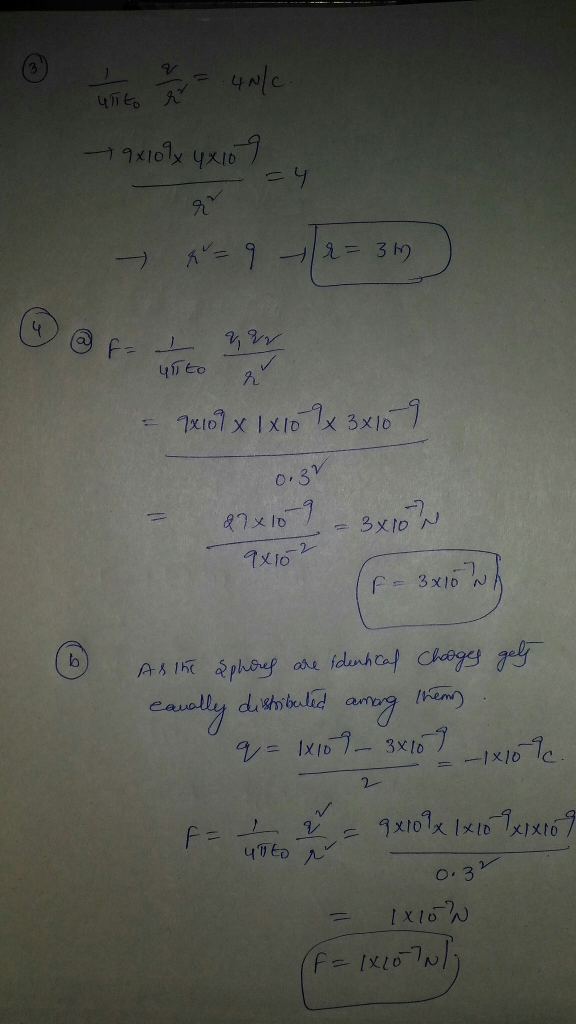#### Earn Coin

Coins can be redeemed for fabulous gifts.

Similar Homework Help Questions
• ### 1. A particle with a charge of +4.20 nC is in a uniform electric field E⃗  directed...

1. A particle with a charge of +4.20 nC is in a uniform electric field E⃗  directed to the negative x direction. It is released from rest, and after it has moved 6.00 cm , its kinetic energy is found to be 1.50×10−6 J . a. What work was done by the electric force? b. What was the change in electric potential over the distance that the charge moved? c. What is the magnitude of E? d. What was the change...

• ### QUESTION 10 Two conducting parallel plates have an electric field between them of magnitude 150 V/m,...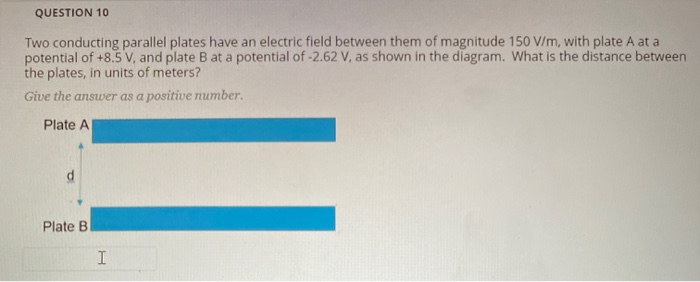QUESTION 10 Two conducting parallel plates have an electric field between them of magnitude 150 V/m, with plate A at a potential of +8.5 V, and plate B at a potential of -2.62 V, as shown in the diagram. What is the distance between the plates, in units of meters? Give the answer as a positive number. Plate A Plate B I QUESTION 9 Two conducting parallel plates have a potential difference of 21.8 V between them. If the electric...

• ### l unnohm electric field. What is the magnitude of the electric field? If this charge is...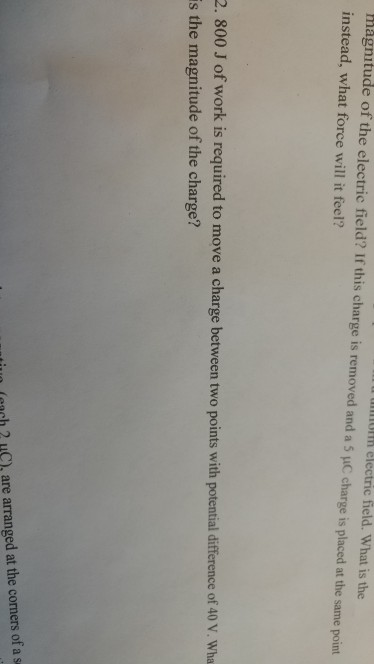l unnohm electric field. What is the magnitude of the electric field? If this charge is removed and a 5 μC charge is placed at the same point instead, what force will it feel? 2. 800 J of work is required to move a charge between two points with potential difference of 40 V. Wha is the magnitude of the charge? iuo (ach 2 HC), are arranged at the corners of a s

• ### Problems 4-9 refer to this same capacitor: The plates of a parallel plate capacitor each have...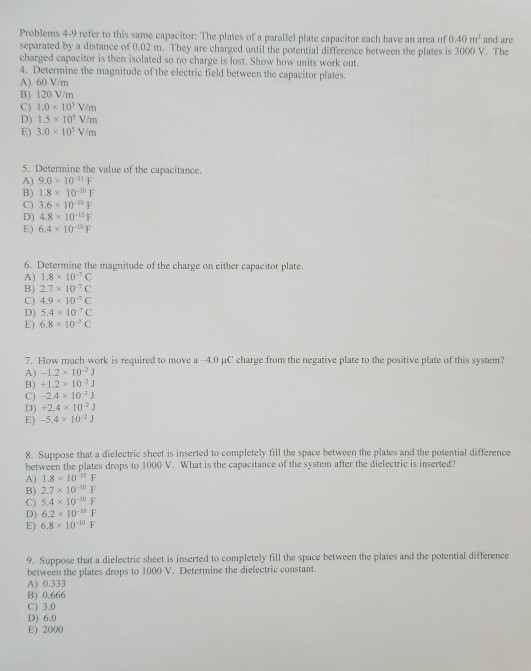Problems 4-9 refer to this same capacitor: The plates of a parallel plate capacitor each have an area of 0.40 m and are separated by a distance of 0.02 m. They are charged until the potential difference between the plates is 3000 V. The charged capacitor is then isolated so no charge is lost. Show how units work out. 4. Determine the magnitude of the electric field between the capacitor plates. A) 60 V/m B) 120 V/m C) 1.0 x...

• ### pgtual and inverted 6. The charge on each plate of a parallel-plate capacitor is 20 uC. The capacitance of the capacitor is C. 1 HF 10-6 F. 12) What is the magnitude of the electric field betwee...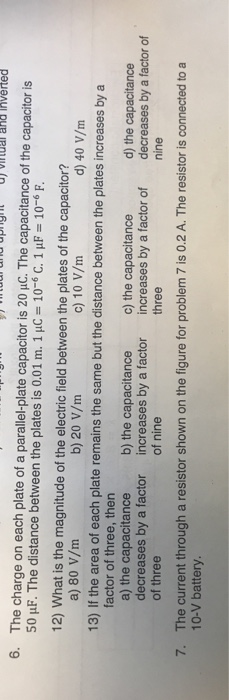pgtual and inverted 6. The charge on each plate of a parallel-plate capacitor is 20 uC. The capacitance of the capacitor is C. 1 HF 10-6 F. 12) What is the magnitude of the electric field between the plates of the capacitor? a) 80 V/m 13) If the area of each plate remains the same but the distance between the plates increases by a factor of three, thern a) the capacitance b) the capacitance c) the capacitance decreases by a...

• ### COI) (15 pts) Two capacitors, C and C2, are connected in series to a 60 V battery as shown in the sketch in part b). The capacitance of C, is 1.0 x10 F, and it carries a charge of2.0 10 C. R-100 ohms...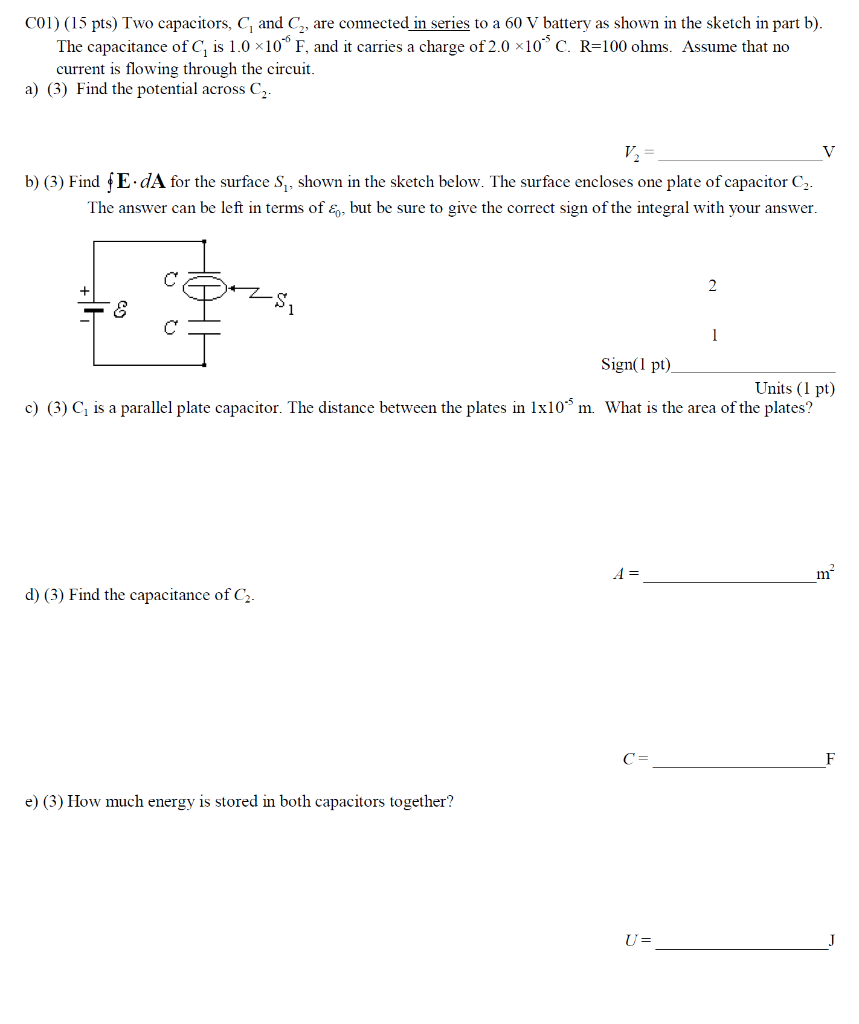COI) (15 pts) Two capacitors, C and C2, are connected in series to a 60 V battery as shown in the sketch in part b). The capacitance of C, is 1.0 x10 F, and it carries a charge of2.0 10 C. R-100 ohms. Assume that no current is flowing through the circuit. a) () Find the potential across C b) (3) Find fE dA for the surface S, shown in the sketch below. The surface encloses one plate of capacitor...

• ### 1) Calculate the magnitude of the electric field 2.12m from a point charge of 6.4mC (such...

1) Calculate the magnitude of the electric field 2.12m from a point charge of 6.4mC (such as found on the terminal of a Van de Graaff). ??NC 2) The electric field strength between two parallel conducting plates separated by 7.0cm is 8.5x10^4 V/m. The potential difference between the plates is 6kV. The with the lowest potential is taken to he zero volts. What is the potential 1.1cm from that plate?

• ### Electric Field / Electric Potentiel / Electric Current

1) A 5.0-C charge is 10 m from a small test charge. What is the magnitude of the electric field at the location of the test charge?2)Two parallel plates, separated by 0.20 m, are connected to a 12-V battery. An electron released from rest at a location 0.10 m from the negative plate. When theelectron arrives at a distance 0.050 m from the positive plate, how much kinetic energy does the electron gain?3)A 4.0-g object carries a charge of 20...

• ### astatements about electric potential and electric potential energy is/are correct? he electric potential energy ap...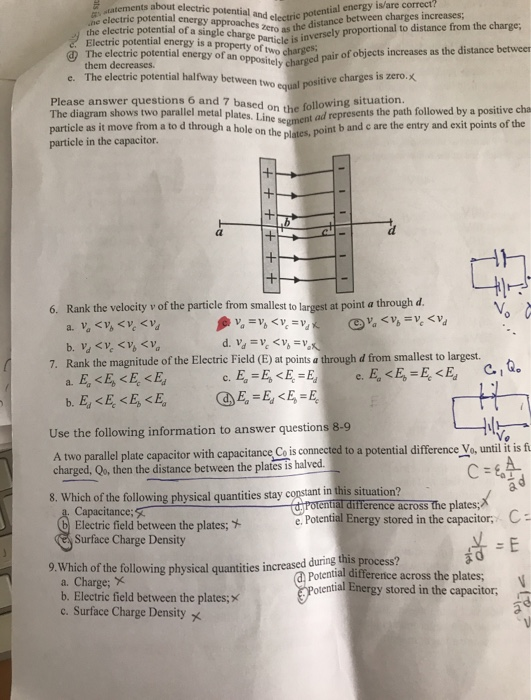astatements about electric potential and electric potential energy is/are correct? he electric potential energy approaches zero as the distance between charges increases; the electric potential of a single charge particle is inversely proportional to distance from the charge, Electric potential energy is a property of two charges The electric potential energy of an oppositely charged pair of objects increases as the distance betweem e. The electric potential halfway between two equal positive charges is zero.x them decreases. Please answer questions...

• ### Problem 5 In the figure below C1 2.0 μF, G 10.0pE, C3 3,0 μF , C.-6.0 μF.G-4.0 μ. The applied potential difference between a and b is Vab 10V. a) [4 points] Calculate the equivalent capacitance o...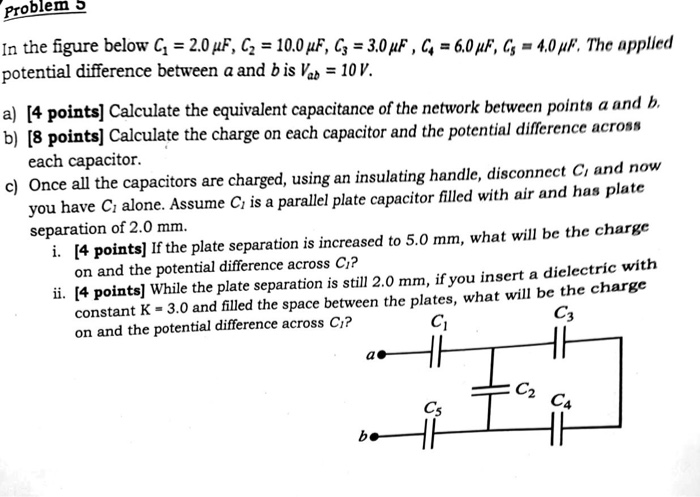Problem 5 In the figure below C1 2.0 μF, G 10.0pE, C3 3,0 μF , C.-6.0 μF.G-4.0 μ. The applied potential difference between a and b is Vab 10V. a) [4 points] Calculate the equivalent capacitance of the network between points a and b. b) [8 pointsl Calculate the charge on each capacitor and the potential difference across each capacitor. c) Once all the capacitors are charged, using an insulating handle, disconnect Ci and now you have C alone. Assume...

Free Homework App